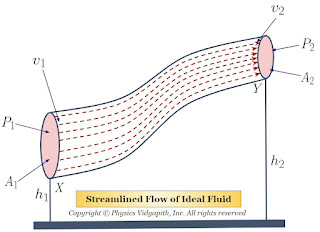## Difference between sound waves and light waves

Sound Waves:

• Sound waves are mechanical waves in nature.
• They need a medium to propagate and so cannot be produced in a vacuum.
• They can move in all types of mediums as solid liquid or gas whether they are transparent or not.
• They propagate in the form of longitudinal waves.
• The particle of the medium vibrates along the direction of the propagation and so contraction and rarefaction are formed there.
• These are three-dimensional waves.
• These waves do not show a polarization effect.
• For a normal human being the audible frequency range is $20$ to $20000 Hertz$.
• The speed of the sound waves is more in a dense medium than in a rare medium.

• Light waves:

• light waves are electromagnetic waves in nature.
• They don't need any medium and so can produce and propagate in a vacuum.
• Their velocity in a vacuum is the maximum of value $3 \times 10^{8} m/s$
• They can move in a transparent medium only.
• They propagate in the form of transverse waves.
• The electric field and magnetic field vibration are perpendicular to the direction of wave propagation.
• These wave waves are also three-dimensional waves.
• These waves source the polarization effect.
• For a normal human being the visible frequency range is $4 \times 10^{18} Hz$ to $8 \times 10^{14} Hz$.
• The speed of light is more in rare mediums than in dense ones.
• ## Popular Posts

### Bernoulli's Theorem and Derivation of Bernoulli's EquationStatement of Bernoulli's Theorem: When an ideal fluid (i.e incompressible and non-viscous Liquid or Gas) flows in streamlined motion from one place to another, then the total energy per unit volume (i.e Pressure energy + Kinetic Energy + Potential Energy) at each and every of its path is constant. $P+\frac{1}{2}\rho v^{2} + \rho gh= constant$ Derivation of Bernoulli's Theorem Equation: Let us consider that an incompressible and non-viscous liquid is flowing in streamlined motion through a tube $XY$ of the non-uniform cross-section. Now Consider: The Area of cross-section $X$ = $A_{1}$ The Area of cross-section $Y$ = $A_{2}$ The velocity per second (i.e. equal to distance) of fluid at cross-section $X$ = $v_{1}$ The velocity per second (i.e. equal to distance) of fluid at cross-section $Y$ = $v_{2}$ The Pressure of fluid at cross-section $X$ = $P_{1}$ The Pressure of fluid at cross-section $Y$ = $P_{2}$ The height of cross-section $X$ from